# Data Science

Data science is a broad field that refers to the collective processes, theories, concepts, tools and technologies that enable the review, analysis and extraction of valuable knowledge and information from raw data.1627279971

## Why I Quit Data Science

Software developer Sufyan Khot’s LinkedIn post titled ‘I quit data science’ sparked a lively debate on the platform. At the time of writing this article, the post had garnered close to 1,400 ‘likes’ and over 90 comments–a testament to how the post struck a chord with a large number of people.

#data-science #datascientist1627270647

## 8 Scala Libraries For Data Science In 2021

Programming language Scala combines object-oriented and functional programming. It is an extension of Java and runs on Java Virtual Machine (JVM). Many developers prefer Scala over Java since the same programmes can be written on the former using a significantly smaller number of lines.

https://analyticsindiamag.com/eight-scala-libraries-for-data-science-in-2021/

#data-science1627224778

## Top 3 Free Machine Learning Courses for Beginners

In this video I talk about my favorite free Machine Learning Crash Courses.

Course 1: Introduction to Machine Learning Problem Framing! This course helps you frame machine learning (ML) problems:

Course 2: Google Machine Learning Crash Course: A self-study guide for aspiring machine learning practitioners. It features a series of lessons with video lectures, real-world case studies, and hands-on practice exercises using TensorFlow:

Course 3: Kaggle’s Intro to Machine Learning and Intermediate Machine Learning: Learn the core ideas in machine learning, and build your first models. Learn how to handle missing values, non-numeric values, data leakage, and more:
https://www.kaggle.com/learn/intro-to-machine-learning
https://www.kaggle.com/learn/intermediate-machine-learning

#machine-learning #data-science #developer1627186575

## Hướng Dẫn Các Bước Tiền Xử Lý Dữ Liệu bằng Scikit-Learn

Hello Diu Túp, hôm nay chúng mình xin giới thiệu đến các bạn 1 video mới trong Series “Tự Học Data Science Cho Người Mới Bắt Đầu”. Và chủ đề của Video hôm này đó chính là “Hướng Dẫn Các Bước Tiền Xử Lý Dữ Liệu bằng Scikit-Learn” 🤩 !

Tiền xử lý dữ liệu (Data Pre-Processing) là một kỹ thuật được sử dụng để chuyển đổi dữ liệu thô thành một định dạng dễ hiểu. Dữ liệu trong thế giới thực (dữ liệu thô) luôn không đầy đủ và dữ liệu đó không thể được gửi qua các mô hình vì nó sẽ gây ra một số lỗi nhất định. Đó là lý do tại sao chúng ta cần xử lý trước dữ liệu trước khi gửi nó qua một mô hình.

⏱ Timestamps:

• [0:00] Giới thiệu về Series “Tự Học Data Science Cho Người Mới Bắt Đầu”
• [0:30] Giới thiệu về Nội Dung của Video
• [5:00] Nhập các thư viện và Đọc tập dữ liệu
• [8:00] Xử lý các giá trị bị thiếu (missing data replacement)
• [20:30] Mã hoá các dữ liệu danh mục
• [27:40] Cách tách dữ liệu (dataset) thành training và test sets
• [40:00] Tiêu chuẩn hóa / Tỷ lệ tính năng (Feature Scaling)

#scikit-learn #data-science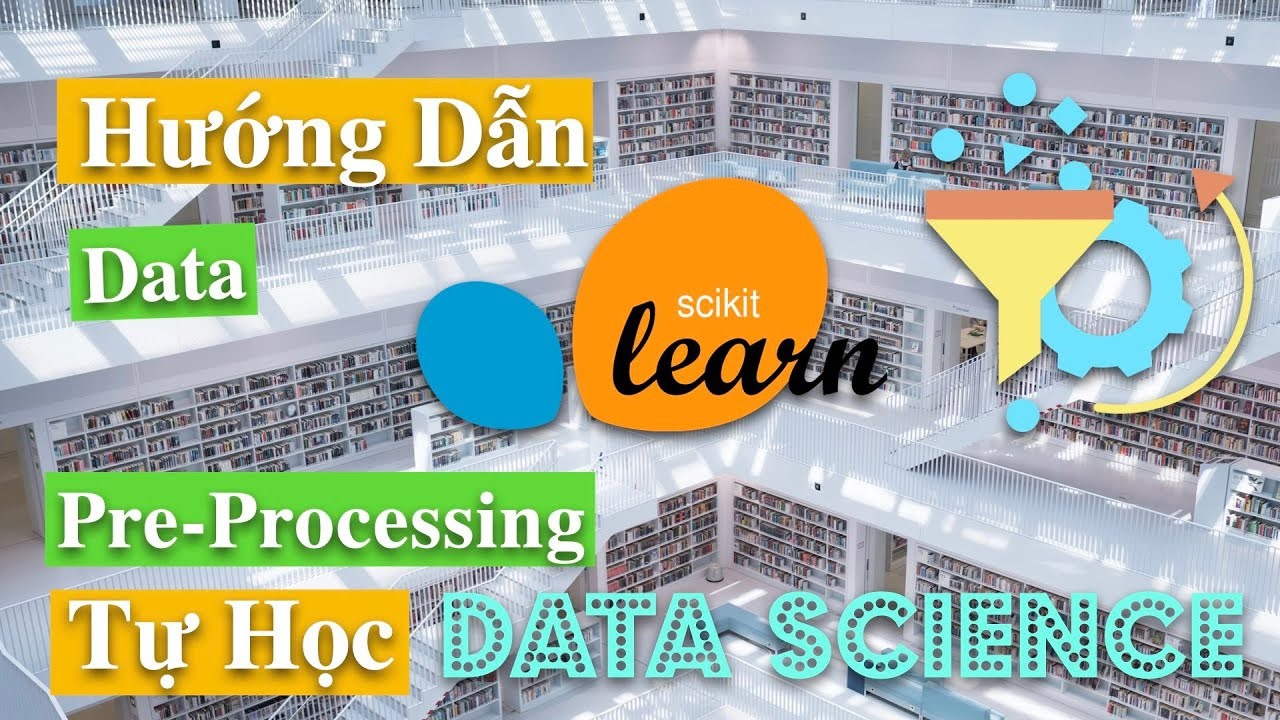1627184046

## Everything You Need to Know About OpenCV Python

OpenCV-Python is a Python library specially designed for solving computer vision problems. OpenCV in Python uses NumPy, another Python library, which adds support for large arrays along with a huge collection of high-level mathematical functions to operate on these arrays. Python is a widely learnt programming language, and face detection is another one of its many popular applications which a lot of people want to learn today. Great Learning brings you this tutorial on OpenCV in Python to help you understand everything you need to know about this topic and getting started on the journey to learn about it well.

• 00:00:00 Introduction
• 00:01:34 Agenda
• 00:03:27 Introduction to OpenCV
• 00:19:26 How to install OpenCV?
• 00:24:12 Process of Computer Vision
• 00:42:25 Hands-on: OpenCV operations
• 00:55:14 What is face detection?
• 01:01:52 What is face recognition?
• 01:09:41 Applications of face recognition
• 01:20:55 Face recognition using Deep Learning
• 01:31:50 Hands-on: Face detection with OpenCV
• 01:44:32 Summary

#opencv #python #data-science #machine-learning1627136040

## The Road to a Serverless ML Pipeline in Production — Part II

### The MLOps architecture we designed and how it’s implemented

In  part 1, I explained the process we went through in creating a fully-automated MLOps architecture, and our decision-making process, considering our needs and the stack we were already using.

In this post, I’ll show you the solution we ended up, including code examples of how to implement this solution yourself.

## Let’s look at the architecture again…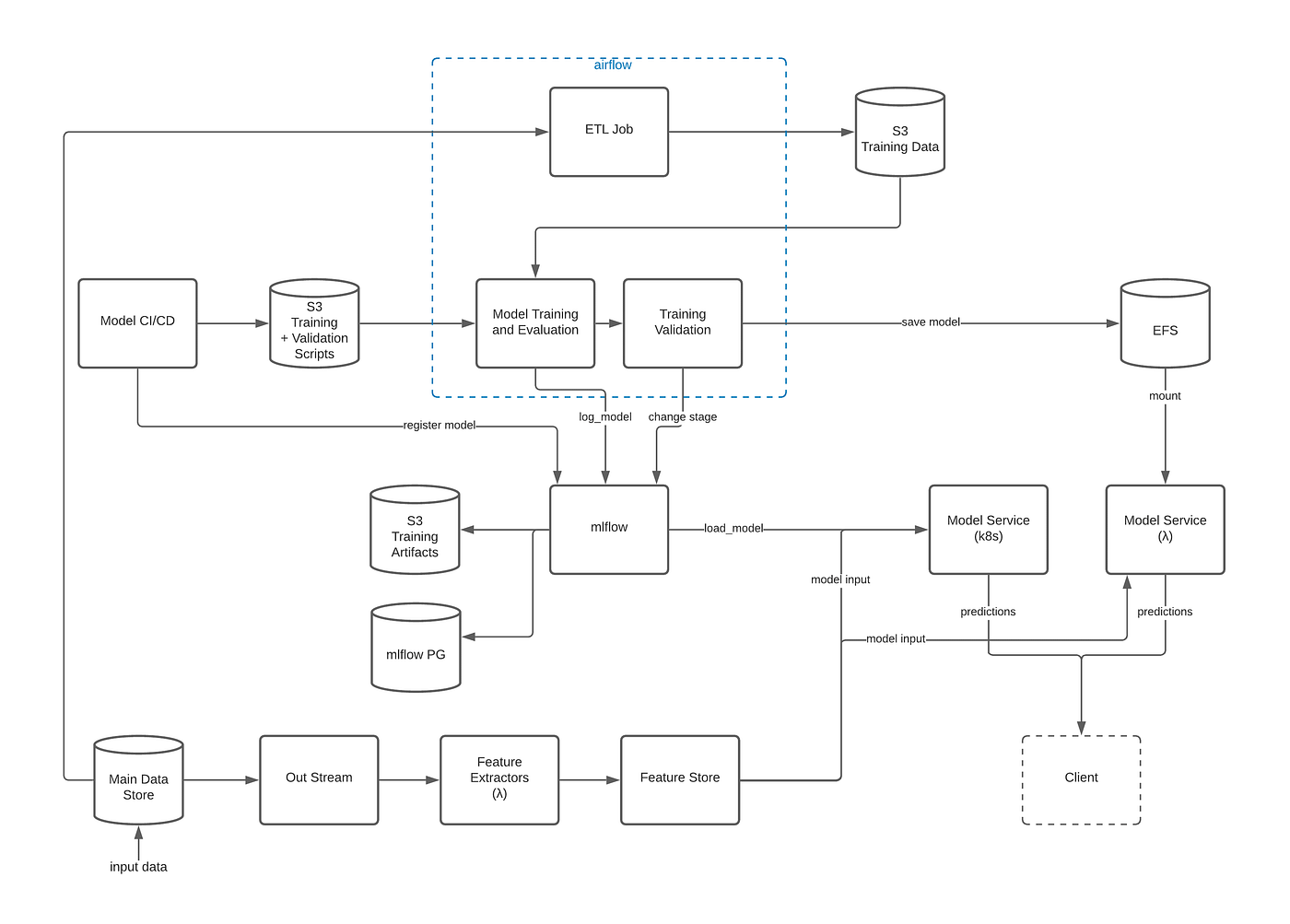Nutrino’s MLOps architecture (Image by Author)

### How does the new pipeline work?

Data scientists work in a research environment on their notebooks (we use Zeppelin) to explore and develop their models. Once they’ve figured out the model they want to write, they go to the model’s project in our source control and start developing the required scripts — the model’s inference service, the training script, and the validation script.

Using Pycharm, the data scientists can work locally (with local Spark) or in front of a remote EMR cluster to run and test their scripts with all the data they’re used to working with in the research environment. They can also easily write unit tests for any of the model parts (serving, training, validation, etc.).

We chose to use git tags to trigger the model’s CI/CD, so that when they put a tag with the new version number — it will trigger the CI/CD process that runs the following:

1. Run all the tests in the project.
2. If passed — copy the training and validation scripts to a specific S3 bucket (referred to hereon as models_bucket) under the path /<model_name>/<version_from_tag>.
3. Deploy the model service.
4. Call mlflow.create_registered_model passing the name for the model as _<model_name>v<version_from_tag> (i.e. my_model_v1).

#data-science #mlops #serverless1627128660

## The Road to a Serverless ML Pipeline in Production — Part I

### How Nutrino designed a serverless MLOps stack in production

Bringing ML models to production today is complicated — different companies have different requirements from the ML stack and there are many tools out there, each tool tries to solve a different aspect of the ML lifecycle. These tools are still a work in progress and there’s no one “clear cut” solution for MLOps. In this article, I’d like to share the process we went through in creating our own MLOps stack, including the way our team worked before the process started, the research we did on different MLOps tools, and how we decided on the solution that fit our non-standard models.

For the detailed solution, including more technical explanations, check out  part II of this article.

TL;DR - We managed to run different types of models (our own Python models) with multiple production versions for each of them — all in a serverless environment!

## Our ML stack pre-refactoring

This was our ML stack before we began the process of refactoring: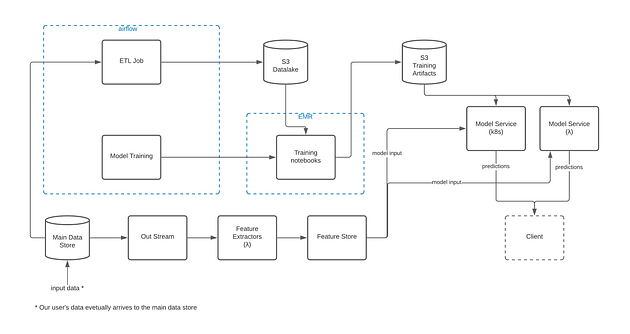Image by Author

### Research Environment

We were using a datalake environment which had an ETL process that transferred production data to parquet files to S3 in that environment. The data scientists were doing their research using Zeppelin notebooks running on EMR clusters in that environment (thus utilizing the distributed abilities of Spark).

### Feature Extraction

Feature extraction was done using AWS lambdas that were triggered by a Kinesis stream every time new data arrived to our centralized data store, and was deployed using the Serverless Framework.

### Training

1. Once a research for a certain model was completed, the data scientist created a training notebook for that model (in the same datalake environment).
2. We ran the training’s notebook periodically using Apache Airflow (and leveraging its strength in running scheduled jobs). To do so, we created a DAG (Directed Acyclic Graph) for each of the models’ training notebooks.
3. Airflow’s DAGs were deployed instantly every push to the master branch.
4. The DAGs created an EMR cluster which ran the training notebooks. The notebooks were connected to a GitHub repository, so that every commit in a notebook was basically an automated “deployment” of the training code.

#mlops #serverless #data-science1627101105

## miceforest: Fast Imputation with Random Forests in Python

### miceforest: Fast Imputation with Random Forests in Python

Fast, memory efficient Multiple Imputation by Chained Equations (MICE) with random forests. It can impute categorical and numeric data without much setup, and has an array of diagnostic plots available. The R version of this package may be found here.

This document contains a thorough walkthrough of the package, benchmarks, and an introduction to multiple imputation. More information on MICE can be found in Stef van Buuren’s excellent online book, which you can find here.

#### Package Meta

miceforest has 4 main classes which the user will interact with:

• `KernelDataSet` - a kernel data set is a dataset on which the mice algorithm is performed. Models are saved inside the instance, which can also be called on to impute new data. Several plotting methods are included to run diagnostics on the imputed data.
• `MultipleImputedKernel` - a collection of `KernelDataSet`s. Has additional methods for accessing and comparing multiple kernel datasets together.
• `ImputedDataSet` - a single dataset that has been imputed. These are returned after `impute_new_data()` is called.
• `MultipleImputedDataSet` - A collection of datasets that have been imputed. Has additional methods for comparing the imputations between datasets.

This package can be installed using either pip or conda, through conda-forge:

``````# Using pip
\$ pip install miceforest

# Using conda
\$ conda install -c conda-forge miceforest
``````

You can also download the latest development version from this repository. If you want to install from github with conda, you must first run `conda install pip git`.

``````\$ pip install git+https://github.com/AnotherSamWilson/miceforest.git
``````

### Using miceforest

We will be looking at a few simple examples of imputation. We need to load the packages, and define the data:

``````import miceforest as mf
import pandas as pd
import numpy as np

# Load data and introduce missing values
iris['target'] = iris['target'].astype('category')
iris_amp = mf.ampute_data(iris,perc=0.25,random_state=1991)
``````

#### Imputing a Single Dataset

If you only want to create a single imputed dataset, you can use `KernelDataSet`:

``````# Create kernel.
kds = mf.KernelDataSet(
iris_amp,
save_all_iterations=True,
random_state=1991
)

# Run the MICE algorithm for 3 iterations
kds.mice(3)

# Return the completed kernel data
completed_data = kds.complete_data()
``````

There are also an array of plotting functions available, these are discussed below in the section Diagnostic Plotting. The plotting behavior between single imputed datasets and multi-imputed datasets is slightly different.

#### Simple Example of Multiple Imputation

We can also create a class which contains multiple `KernelDataSet`s, along with easy ways to compare them:

``````# Create kernel.
kernel = mf.MultipleImputedKernel(
iris_amp,
datasets=4,
save_all_iterations=True,
random_state=1991
)

# Run the MICE algorithm for 3 iterations on each of the datasets
kernel.mice(3)
``````

Printing the `MultipleImputedKernel` object will tell you some high level information:

``````print(kernel)
``````
``````##               Class: MultipleImputedKernel
##        Models Saved: Last Iteration
##            Datasets: 4
##          Iterations: 3
##   Imputed Variables: 5
## save_all_iterations: True

``````

#### Controlling Tree Growth

A very nice thing about random forests is that they are trivially parallelizable. We can save a lot of time by setting the `n_jobs` parameter in both the fit and predict methods for the random forests:

``````# Run the MICE algorithm for 2 more iterations on the kernel
kernel.mice(2,n_jobs=2)
``````

Any other arguments may be passed to either class (`RandomForestClassifier`,`RandomForestRegressor`). In our example, we may not have saved much (if any) time. This is because there is overhead with using multiple cores, and our data is very small.

#### Creating a Custom Imputation Schema

It is possible to customize our imputation procedure by variable. By passing a named list to `variable_schema`, you can specify the predictors for each variable to impute. You can also select which variables should be imputed using mean matching, as well as the mean matching candidates, by passing a dict to`mean_match_candidates`:

``````var_sch = {
'sepal width (cm)': ['target','petal width (cm)'],
'petal width (cm)': ['target','sepal length (cm)']
}
var_mmc = {
'sepal width (cm)': 5,
'petal width (cm)': 0
}

cust_kernel = mf.MultipleImputedKernel(
iris_amp,
datasets=3,
variable_schema=var_sch,
mean_match_candidates=var_mmc
)
cust_kernel.mice(2)
``````

#### Imputing New Data with Existing Models

Multiple Imputation can take a long time. If you wish to impute a dataset using the MICE algorithm, but don’t have time to train new models, it is possible to impute new datasets using a `MultipleImputedKernel` object. The `impute_new_data()` function uses the random forests collected by `MultipleImputedKernel` to perform multiple imputation without updating the random forest at each iteration:

``````# Our 'new data' is just the first 15 rows of iris_amp
new_data = iris_amp.iloc[range(15)]
new_data_imputed = kernel.impute_new_data(new_data=new_data)
print(new_data_imputed)
``````
``````##               Class: MultipleImputedDataSet
##            Datasets: 4
##          Iterations: 5
##   Imputed Variables: 5
## save_all_iterations: False

``````

All of the imputation parameters (variable_schema, mean_match_candidates, etc) will be carried over from the original `MultipleImputedKernel` object. When mean matching, the candidate values are pulled from the original kernel dataset. To impute new data, the `save_models` parameter in `MultipleImputedKernel` must be > 0. If `save_models == 1`, the model from the latest iteration is saved for each variable. If `save_models > 1`, the model from each iteration is saved. This allows for new data to be imputed in a more similar fashion to the original mice procedure.

### Diagnostic Plotting

As of now, miceforest has four diagnostic plots available.

#### Distribution of Imputed-Values

We probably want to know how the imputed values are distributed. We can plot the original distribution beside the imputed distributions in each dataset by using the `plot_imputed_distributions` method of an `MultipleImputedKernel` object:

``````kernel.plot_imputed_distributions(wspace=0.3,hspace=0.3)
``````The red line is the original data, and each black line are the imputed values of each dataset.

#### Convergence of Correlation

We are probably interested in knowing how our values between datasets converged over the iterations. The `plot_correlations` method shows you a boxplot of the correlations between imputed values in every combination of datasets, at each iteration. This allows you to see how correlated the imputations are between datasets, as well as the convergence over iterations:

``````kernel.plot_correlations()
``````#### Variable Importance

We also may be interested in which variables were used to impute each variable. We can plot this information by using the `plot_feature_importance` method.

``````kernel.plot_feature_importance(annot=True,cmap="YlGnBu",vmin=0, vmax=1)
``````The numbers shown are returned from the sklearn random forest `_feature_importance` attribute. Each square represents the importance of the column variable in imputing the row variable.

#### Mean Convergence

If our data is not missing completely at random, we may see that it takes a few iterations for our models to get the distribution of imputations right. We can plot the average value of our imputations to see if this is occurring:

``````kernel.plot_mean_convergence(wspace=0.3, hspace=0.4)
``````Our data was missing completely at random, so we don’t see any convergence occurring here.

### Using the Imputed Data

To return the imputed data simply use the `complete_data` method:

``````dataset_1 = kernel.complete_data(0)
``````

This will return a single specified dataset. Multiple datasets are typically created so that some measure of confidence around each prediction can be created.

Since we know what the original data looked like, we can cheat and see how well the imputations compare to the original data:

``````acclist = []
for iteration in range(kernel.iteration_count()+1):
target_na_count = kernel.na_counts['target']
compdat = kernel.complete_data(dataset=0,iteration=iteration)

# Record the accuract of the imputations of target.
acclist.append(
round(1-sum(compdat['target'] != iris['target'])/target_na_count,2)
)

# acclist shows the accuracy of the imputations
# over the iterations.
print(acclist)
``````
``````## [0.32, 0.76, 0.78, 0.81, 0.86, 0.86]

``````

In this instance, we went from a ~32% accuracy (which is expected with random sampling) to an accuracy of ~86%. We managed to replace the missing `target` values with a pretty high degree of accuracy!

### The MICE Algorithm

Multiple Imputation by Chained Equations ‘fills in’ (imputes) missing data in a dataset through an iterative series of predictive models. In each iteration, each specified variable in the dataset is imputed using the other variables in the dataset. These iterations should be run until it appears that convergence has been met.This process is continued until all specified variables have been imputed. Additional iterations can be run if it appears that the average imputed values have not converged, although no more than 5 iterations are usually necessary.

#### Common Use Cases

##### Data Leakage:

MICE is particularly useful if missing values are associated with the target variable in a way that introduces leakage. For instance, let’s say you wanted to model customer retention at the time of sign up. A certain variable is collected at sign up or 1 month after sign up. The absence of that variable is a data leak, since it tells you that the customer did not retain for 1 month.

##### Funnel Analysis:

Information is often collected at different stages of a ‘funnel’. MICE can be used to make educated guesses about the characteristics of entities at different points in a funnel.

##### Confidence Intervals:

MICE can be used to impute missing values, however it is important to keep in mind that these imputed values are a prediction. Creating multiple datasets with different imputed values allows you to do two types of inference:

• Imputed Value Distribution: A profile can be built for each imputed value, allowing you to make statements about the likely distribution of that value.
• Model Prediction Distribution: With multiple datasets, you can build multiple models and create a distribution of predictions for each sample. Those samples with imputed values which were not able to be imputed with much confidence would have a larger variance in their predictions.

#### Predictive Mean Matching

`miceforest` can make use of a procedure called predictive mean matching (PMM) to select which values are imputed. PMM involves selecting a datapoint from the original, nonmissing data which has a predicted value close to the predicted value of the missing sample. The closest N (`mean_match_candidates` parameter) values are chosen as candidates, from which a value is chosen at random. This can be specified on a column-by-column basis. Going into more detail from our example above, we see how this works in practice:This method is very useful if you have a variable which needs imputing which has any of the following characteristics:

• Multimodal
• Integer
• Skewed

#### Effects of Mean Matching

As an example, let’s construct a dataset with some of the above characteristics:

``````randst = np.random.RandomState(1991)
# random uniform variable
nrws = 1000
uniform_vec = randst.uniform(size=nrws)

def make_bimodal(mean1,mean2,size):
bimodal_1 = randst.normal(size=nrws, loc=mean1)
bimodal_2 = randst.normal(size=nrws, loc=mean2)
bimdvec = []
for i in range(size):
bimdvec.append(randst.choice([bimodal_1[i], bimodal_2[i]]))
return np.array(bimdvec)

# Make 2 Bimodal Variables
close_bimodal_vec = make_bimodal(2,-2,nrws)
far_bimodal_vec = make_bimodal(3,-3,nrws)

# Highly skewed variable correlated with Uniform_Variable
skewed_vec = np.exp(uniform_vec*randst.uniform(size=nrws)*3) + randst.uniform(size=nrws)*3

# Integer variable correlated with Close_Bimodal_Variable and Uniform_Variable
integer_vec = np.round(uniform_vec + close_bimodal_vec/3 + randst.uniform(size=nrws)*2)

# Make a DataFrame
dat = pd.DataFrame(
{
'uniform_var':uniform_vec,
'close_bimodal_var':close_bimodal_vec,
'far_bimodal_var':far_bimodal_vec,
'skewed_var':skewed_vec,
'integer_var':integer_vec
}
)

# Ampute the data.
ampdat = mf.ampute_data(dat,perc=0.25,random_state=randst)

# Plot the original data
import seaborn as sns
import matplotlib.pyplot as plt
g = sns.PairGrid(dat)
g.map(plt.scatter,s=5)
``````We can see how our variables are distributed and correlated in the graph above. Now let’s run our imputation process twice, once using mean matching, and once using the model prediction.

``````kernelmeanmatch <- mf.MultipleImputedKernel(ampdat,mean_match_candidates=5)
kernelmodeloutput <- mf.MultipleImputedKernel(ampdat,mean_match_candidates=0)

kernelmeanmatch.mice(5)
kernelmodeloutput.mice(5)
``````

Let’s look at the effect on the different variables.

###### With Mean Matching
``````kernelmeanmatch.plot_imputed_distributions(wspace=0.2,hspace=0.4)
``````###### Without Mean Matching
``````kernelmodeloutput.plot_imputed_distributions(wspace=0.2,hspace=0.4)
``````You can see the effects that mean matching has, depending on the distribution of the data. Simply returning the value from the model prediction, while it may provide a better ‘fit’, will not provide imputations with a similair distribution to the original. This may be beneficial, depending on your goal.

Author: AnotherSamWilson
Official Website: https://github.com/AnotherSamWilson/miceforest

#algorithms #python #data-science1627090600

## AI Engineering Full Course | Artificial Intelligence Full Course

This Artificial Intelligence Engineer course will help us understand the basics of artificial intelligence and the different algorithms used to build AI models. This Artificial Intelligence course will be a great kickstart for all the aspiring AI Engineers.

#artificial-intelligence #ai #data-science #developer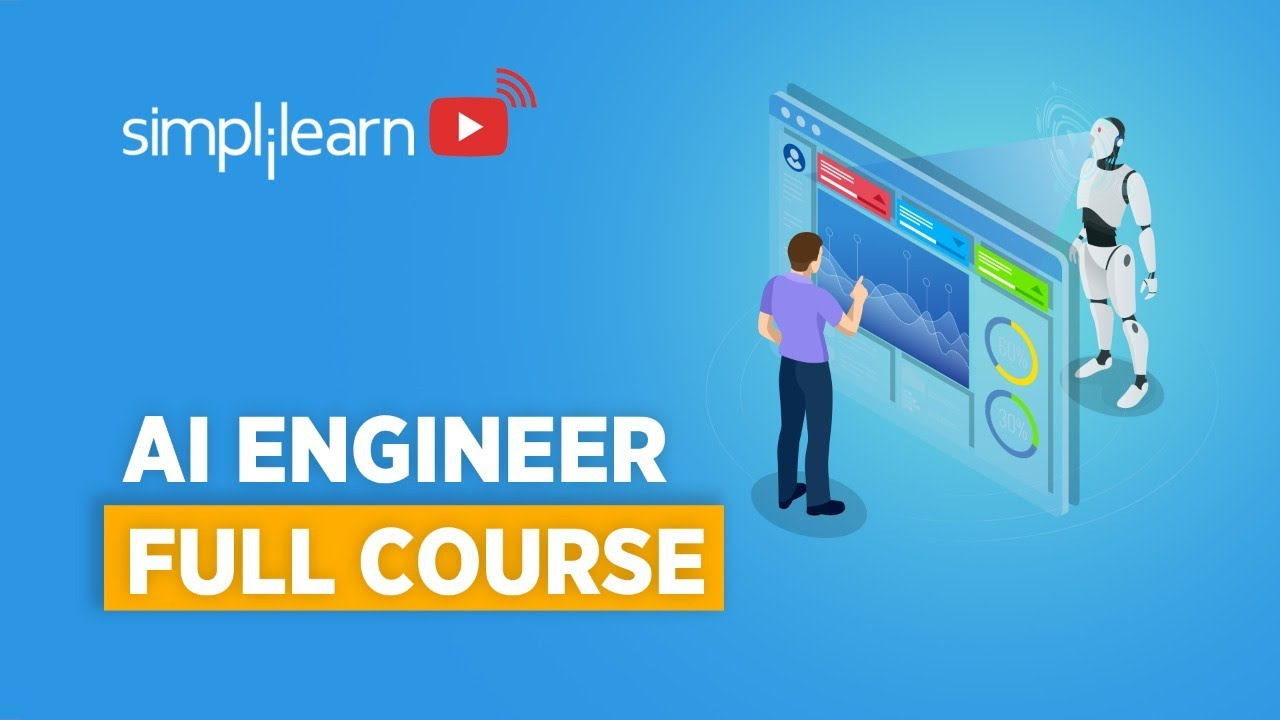1627084206

## Soft Actor Critic (SAC) in Tensorflow2

The Soft Actor Critic Algorithm is a powerful tool for solving cutting edge deep reinforcement learning problems involving continuous action space environments. It’s a variation of the actor critic method that leverages a maximum entropy framework, double Q networks, and target value networks.

The entropy is modeled by scaling the reward factor, with an inverse relationship between the reward scale and the entropy of our agent. Larger reward scaling means more deterministic behavior, and a larger reward scale means more stochastic behavior.

We’re going to implement this algorithm using the tensorflow 2 framework, and test it out on the Inverted Pendulum environment found in the PyBullet package.

https://youtu.be/YKhkTOU0l20

#deep-learning #machine-learning #artificial-intelligence #python #reinforcement-learning #data-science1627073160

## The Ultimate Guide to Functional Programming for Big Data

### Pure Functions and Lazy Evaluations — The Crux of Distributed Data Computations

Apache Spark has become the most commonly used tool in the Big Data universe today. It has the capability of running solo code, extending APIs to Python, Scala, Java, and many more tools. It can be used to query datasets, and the most inspiring part of its architecture is the capability of running analysis on Real-Time Streaming data without explicitly storing it anywhere. Spark originated from Scala and was designed as a distributed cluster-computing software framework. From resource management, multithreading, task distribution to actually running the logic, Spark does everything under the hood. From an end-user perspective, it is an analysis tool where huge amounts of data can be fed and required analyses can be drawn within minutes. But, how does Spark achieve it? What are some core principles of using Spark to work with large datasets?

To ramp up on the basics of Spark, its architecture, and implementation in the Big Data and Cloud world, refer to the story linked below.

#spark #data-science #data #big-data #functional-programming1627053216

## How to Run Object Detection on a Drone

In this video we are going to learn how to run object detection on a drone. We will first look at object detection and then embed it to the drone. And no we not going to install a 100 packages with 50 parameter configurations. You will have your model running it 10 to 15 mins.

#opencv #machine-learning #data-science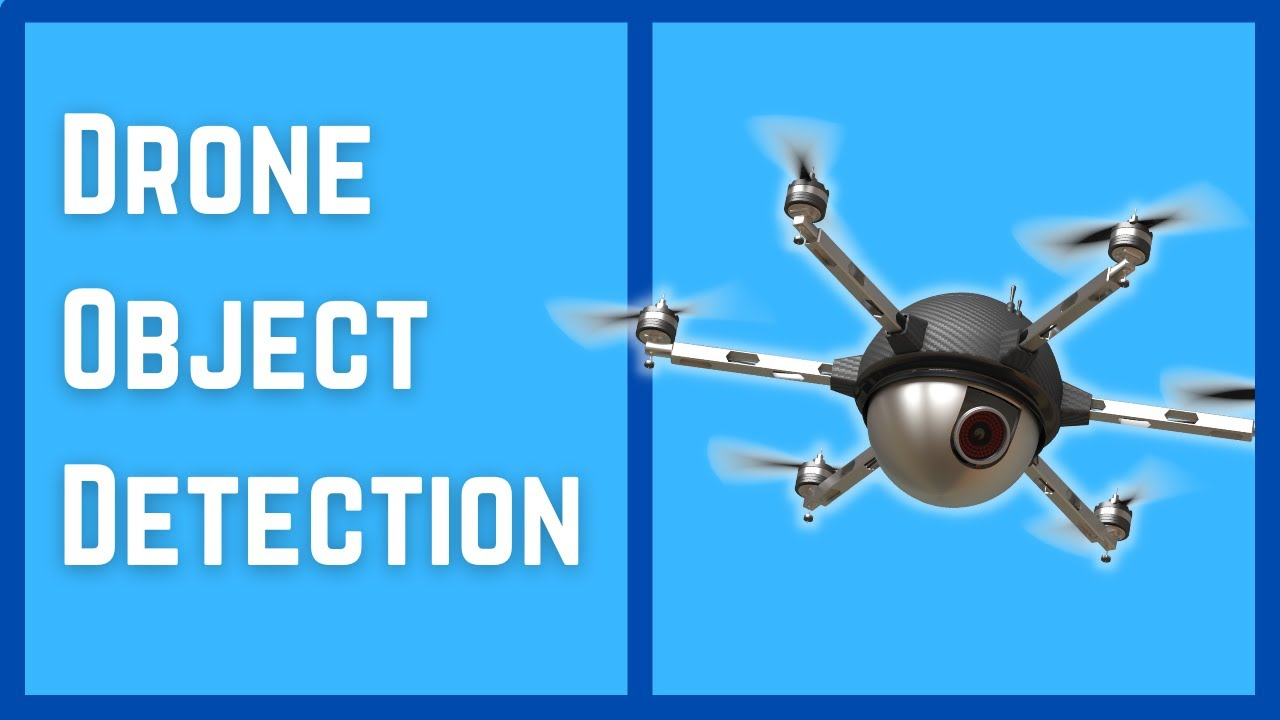1627052100

## Scraping Images Using Selenium

In this small and simple use-case, we explore how to use Selenium to scrap images from Google Chrome for any keyword (or set of keywords) searched by a user.

## Aim

• Our program should take any keyword (For Example: “cat”) from the user, along with the number of images needed, and scrap that many images from Google Images on the Chrome browser.
• The images must be stored in a folder, named after the search term, and should be numbered properly so as to make them easy to access and interpret.

## Program Flow

1. Firstly, our program should accept a search term and number of images that are to be scraped.
2. The program should check if a folder with the same name as the search term already exists or not. If it doesn’t then it should create one.
3. Our program should then open the Google Images page for that particular search term.
4. It should then click on each image and extract its URL which can be used for scraping and saving the image later. It should extract as many URLs as specified.
5. If the webpage doesn’t have that many images, it should click on the “Load More” button to go to the next page
6. Once the list of URLs is created, the program should hit every URL, download the image there and save it in the folder created.

#data-science #web-scraping #scraping #selenium #machine-learning1627050540

## Big Data — Know Your Data

Big data is less about size, and more about how we understand data. In order to better understand data, first we need to understand the problem.

1. So the first step is to accurately identify the problem we want to target — What is the business problem? Why does the problem have to be addressed? Which value do we expect to get when we solve it?
2. Second, we need to fix objectives — What happened in the past and why did it happen? What is happening now, and what should I do in the present? And what do we expect to do in the future, along with our expectations.
3. Identify stake-holders — Technology team, Data scientists, Subject matter experts.

#big-data #data-engineering #data-science1627036740

## Web Scraping with Selenium in Python

Often, data is publicly available to us, but not in a form that is readily useable. That is where web scraping comes in. Web scraping is the process of extracting data from a website. We can use web scraping to get our desired data into a convenient format that can then be used. In this tutorial, I will show how you can extract information of interest from a website using the selenium package in Python. Selenium is extremely powerful. It allows us to drive a browser window and interact with the website programmatically. Selenium also has several methods which make extracting data very easy.

In this tutorial I will be developing in a Jupyter Notebook using Python3 on Windows 10.